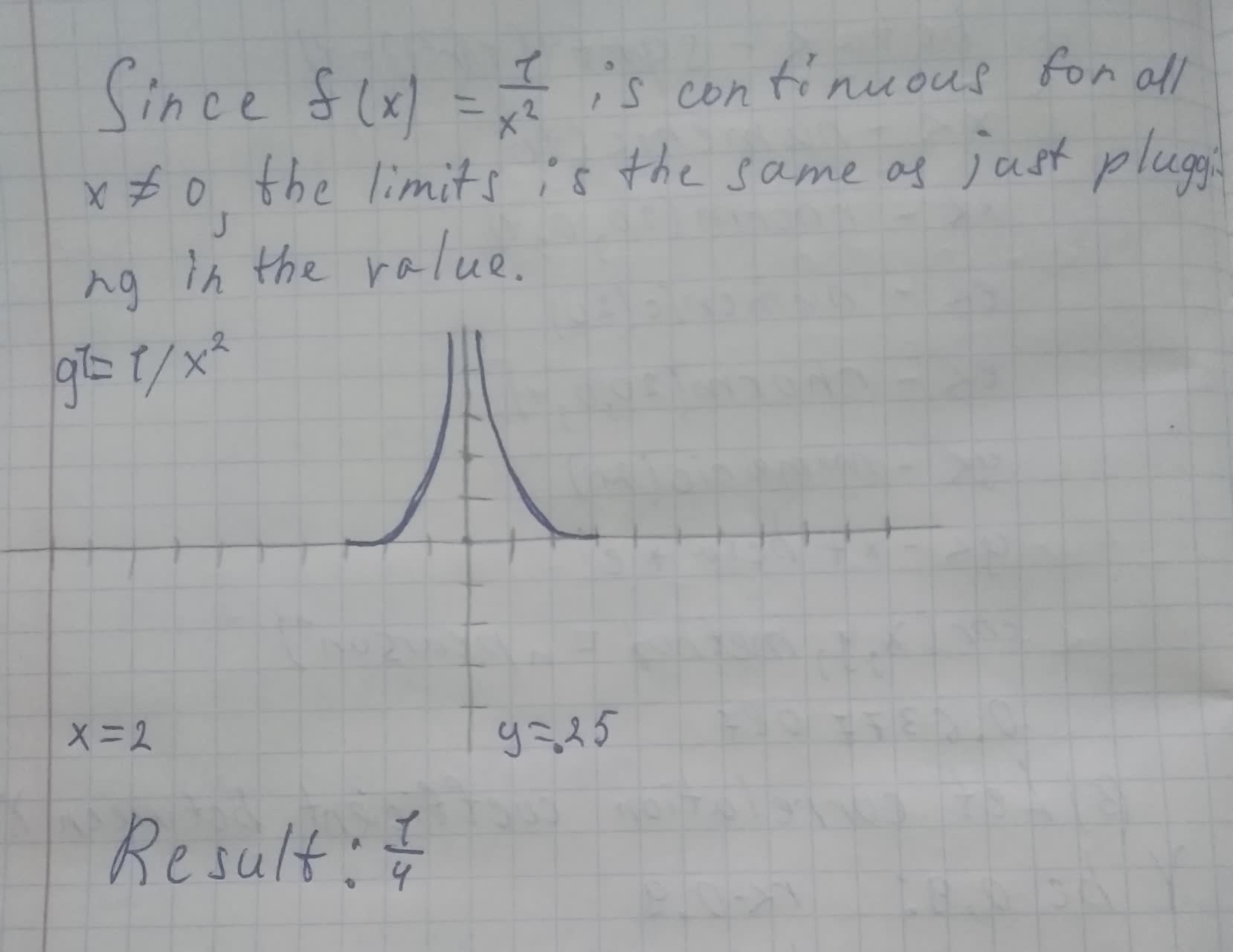Question# The reduced row echelon form of a system of linear equations is given.Write the system of equations corresponding to the given matrix. Use x, y. or x, y, z. or x_1, x_2, x_3, x_4 as variables.

Forms of linear equations
ANSWEREDThe reduced row echelon form of a system of linear equations is given.Write the system of equations corresponding to the given matrix. Use x, y. or x, y, z. or $$x_1, x_2, x_3, x_4$$ as variables. Determine whether the system is consistent or inconsistent. If it is consistent, give the solution.
$$\begin{bmatrix}1 & 0 & 0 & 0 & 1\\ 0 &1 & 0 &0 & 2 \\ 0 & 0 & 1 & 2 & 3 \end{bmatrix}$$